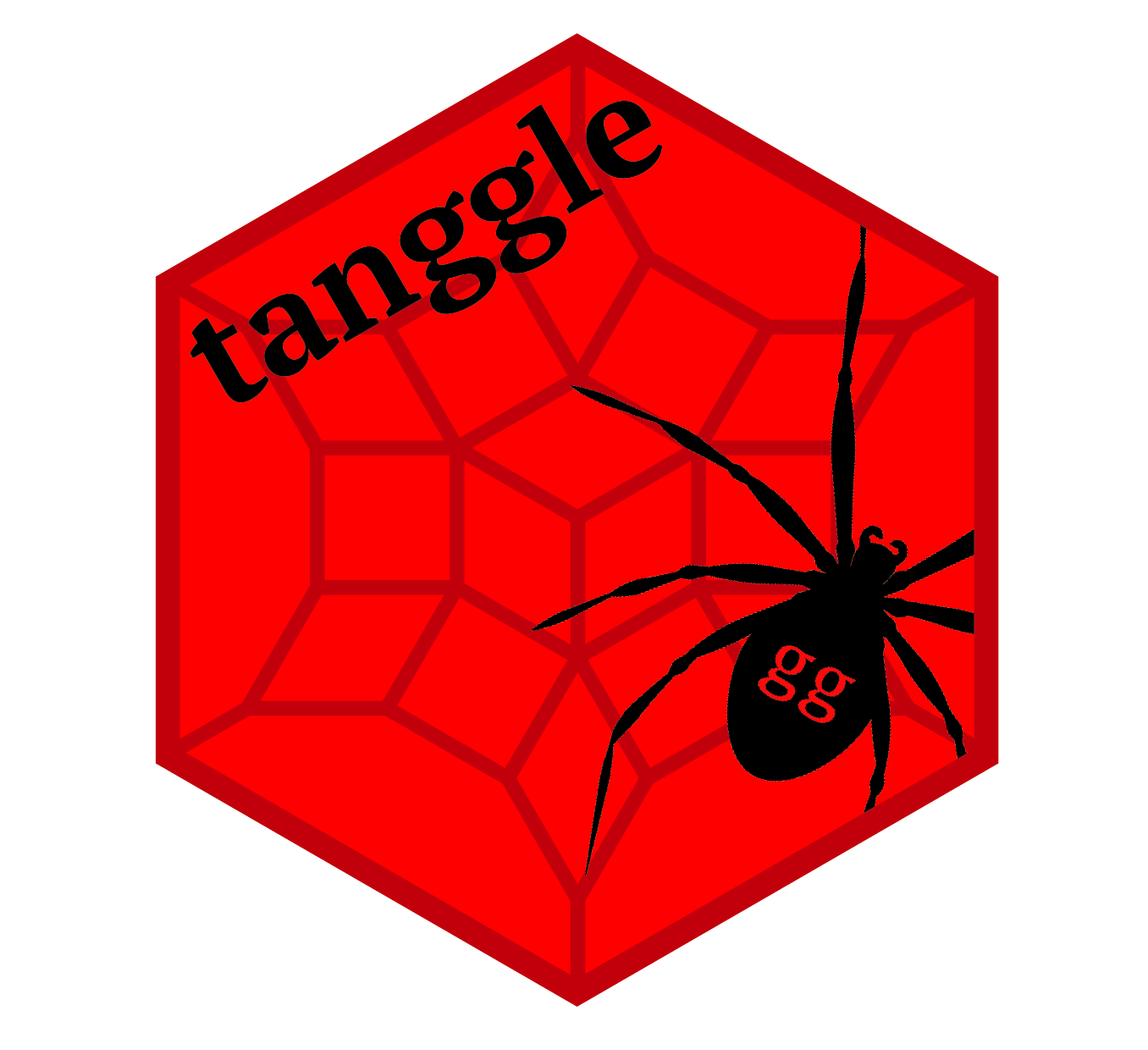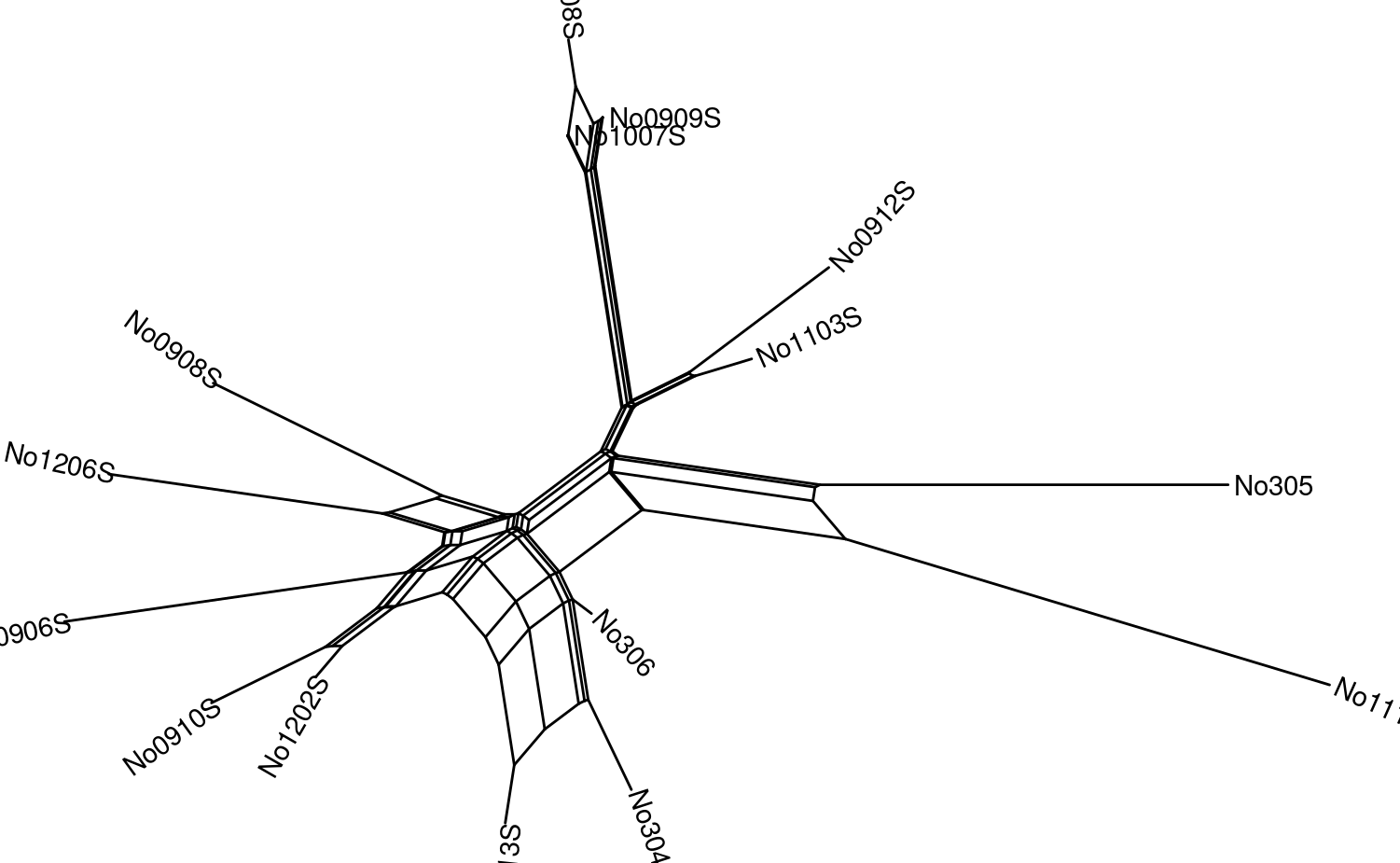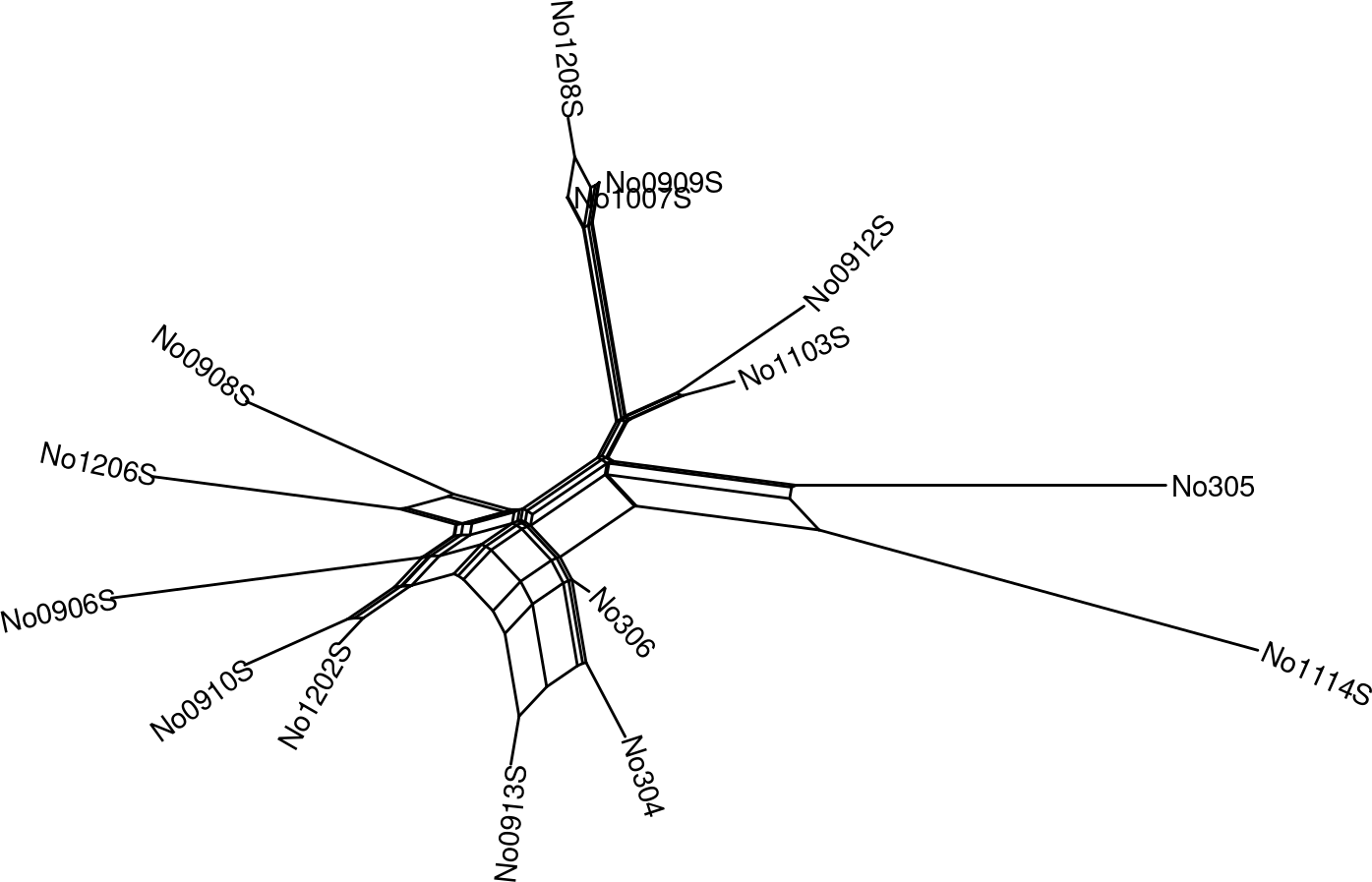# Contents# 1 Introduction

Here we present a vignette for the R package tanggle, and provide an overview of its functions and their usage. Tanggle extends the ggtree R package (Yu et al. 2017) to allow for the visualization of several types of phylogenetic networks using the ggplot2 (Wickham 2016) syntax. More specifically, tanggle contains functions to allow the user to effectively plot: (1) split (i.e. implicit) networks (unrooted, undirected) and (2) explicit networks (rooted, directed) with reticulations. It offers an alternative to the plot functions already available in ape (Paradis and Schliep 2018) and phangorn (Schliep 2011).

# 2 List of functions

Function name Brief description
`geom_splitnet` Adds a splitnet layer to a ggplot, to combine visualising data and the network
`ggevonet` Plots an explicit network from a phylo object
`ggsplitnet` Plots an implicit network from a phylo object
`minimize_overlap` Reduces the number of reticulation lines crossing over in the plot
`node_depth_evonet` Returns the depths or heights of nodes and tips in the phylogenetic network

# 3 Getting started

Install the package from Bioconductor directly:

``````if (!requireNamespace("BiocManager", quietly = TRUE))
install.packages("BiocManager")

BiocManager::install("tanggle")``````

Or install the development version of the package from Github.

``````if (!requireNamespace("remotes", quietly=TRUE))
install.packages("remotes")
remotes::install_github("KlausVigo/tanggle")``````

If you need to install ggtree from github:

``remotes::install_github("YuLab-SMU/ggtree")``

``````library(tanggle)
library(phangorn)
library(ggtree)``````

# 4 Split Networks

Split networks are data-display objects which allow for the definition of 2 (or more) options for non-compatible splits. Split networks are most often used to visualize consensus networks (Holland et al. 2004) or neighbor-nets (Bryant and Moulton 2004). This can be done either by using the `consensusNet` or `neighbor-net` functions in phangorn (Schliep 2011) or by importing nexus files from SplitsTree (Huson and Bryant 2006).

## 4.1 Data Types

tanggle accepts three forms of input data for split networks. The following input options all generate a networx object for plotting.

• Nexus file created with SplitsTree (Huson and Bryant 2006) and read with the `read.nexus.network` function in phangorn (Schliep 2011).

• Read in a split network in nexus format:

``````fdir <- system.file("extdata/trees", package = "phangorn")
1. A collection of gene trees (e.g.~from RAxML (Stamatakis 2014)) in one of the following formats:
• Nexus file read with the function `read.nexus`
• Text file in Newick format (one gene tree per line) read with the function `read.tree` A consensus split network is then computed using the function `consensusNet` in phangorn (Schliep 2011).
• Sequences in nexus, fasta or phylip format, read with the function `read.phyDat` in phangorn (Schliep 2011) or the function `read.dna` in ape (Paradis and Schliep 2018). Distances matrices are then computed for specific models of evolution using the function `dist.ml` in phangorn (Schliep 2011) or `dist.dna` in ape (Paradis and Schliep 2018). From the distance matrix, a split network is reconstructed using the function `neighborNet` in phangorn (Schliep 2011). Optional: branch lengths may be estimated using the function `splitsNetworks` in phangorn (Schliep 2011).

## 4.2 Plotting a Split Network:

We can plot the network with the default options:

``````p <- ggsplitnet(Nnet) + geom_tiplab2()
p``````When we can set the limits for the x and y axis so that the labels are readable.

``````p <- p + xlim(-0.019, .003) + ylim(-.01,.012)
p``````You can rename tip labels. Here we changed the names to species from 1 to 15:

``Nnet\$translate\$label <- seq_along(Nnet\$tip.label)``

We can include the tip labels with `geom_tiplab2`, and customize some of the options. For example, here the tip labels are in blue and both in bold and italics, and we show the internal nodes in green:

``````ggsplitnet(Nnet) + geom_tiplab2(col = "blue", font = 4, hjust = -0.15) +
geom_nodepoint(col = "green", size = 0.25)``````